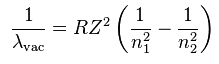# Rydberg Formula for unielectronic species Calculator

This CalcTown Calculator calculates the wavelength and frequency generated by quantum leap of an electron in any hydrogen-like atom according to the Rydberg formula.

#### Result

nm
Hzwhere

λvac= wavelength of light in vacuum

ni= initial quantum state

ni=final quantum state

R=Rydberg constant